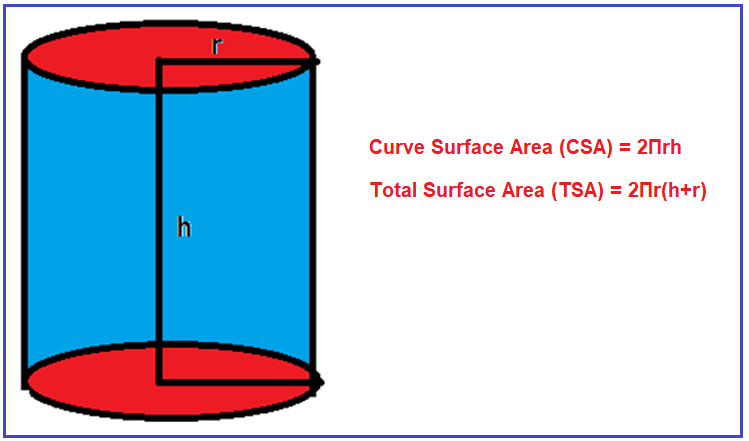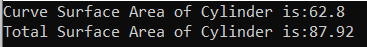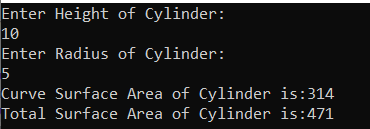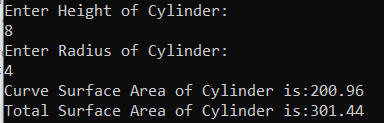# Surface Area of Cylinder in C#

## Surface Area of Cylinder in C# with Examples

In this article, I am going to discuss how to calculate the Curve and Total Surface Area of the Cylinder in C# with Examples. Please read our previous article, where we discussed How to Calculate the Volume of Cuboid in C#. Here, in this article, first, we will discuss what is Cylinder, then we will discuss how to calculate the Surface Area of the Cylinder and finally, we will see how to implement the Surface Area of the Cylinder program in C#.

##### What is a Cylinder?

A cylinder in mathematics is a three-dimensional solid figure which consists of two circular bases connected with two parallel lines. two parallel lines form a curved surface that is bounded by two circles at the top and bottom.

The Surface area of a cylinder is the number of square units that will exactly cover the outer surface of a cone. There are three surfaces in a cylinder, one curved and two circular bases. The total surface area of a cylinder is the sum of the area of both circular bases and the area of the curved surfaces. The total surface area includes the area of the circular top and base, as well as the Curved Surface Area(CSA).

We can calculate the curved surface area and total surface area by using the following formula:
Curve Surface Area (CSA) = 2Πrh
Total Surface Area (TSA) = 2Πr(h+r)
where r is the radius of the cylinder and h is the height of the cylinder and Π is the constant value i.e. 3.14 or 22/7. For better understanding, please have a look at the following diagram.Example:

Output:
Curve Surface Area = 2πrh = 2x 3.14 x 2 x5
CSA = 62.8

Total Surface Area = 2πr (h + r) = 2x 3.14 x 2 x (5 + 2)
TSA = 87.92

##### Logic to Calculate the Surface Area of Cylinder in C#

To find the Surface Area of the Cylinder, we need to follow the below steps

1. We will define the radius, height and CurveSurfaceArea, and TotalSurfaceArea variables
2. Then we will assign the value of radius and height
3. Then we will calculate the surface area of the cylinder by using the formula. Total Surface Area = 2πr (h + r) and Curve Surface Area = 2πrh
4. And the resulting output will store in CurveSurfaceArea and TotalSurfaceArea variables
##### Example: Surface Area of Cylinder Program in C#.

The following C# Program will calculate the Surface Area of the Cylinder.

```using System;
class AreaOfCylinder
{
static void Main()
{
double PI = 3.14;
Height = 5;

//CSA = 2πrh
CurveSurfaceArea = 2 * PI * Radius * Height;

//TSA = 2πr (h + r)

Console.WriteLine("Curve Surface Area of Cylinder is:" + CurveSurfaceArea);
Console.WriteLine("Total Surface Area of Cylinder is:" + TotalSurfaceArea);

}
}
```
###### Output:##### Example: Taking input from the user

In the following C# program, we will take the cylinder radius and height from the user and then calculate the Surface Area and print it on the console.

```using System;
class AreaOfCylinder
{
static void Main()
{
double PI = 3.14;
Console.WriteLine("Enter Height of Cylinder:");

//CSA = 2πrh
double CurveSurfaceArea = 2 * PI * Radius * Height;

//TSA = 2πr (h + r)
double TotalSurfaceArea = 2 * PI * Radius * (Height + Radius);

Console.WriteLine("Curve Surface Area of Cylinder is:" + CurveSurfaceArea);
Console.WriteLine("Total Surface Area of Cylinder is:" + TotalSurfaceArea);

}
}
```
###### Output:##### C# Code (Using function)

In the below example, we have created two methods to calculate the curve and total surface area.

```using System;
class AreaOfCylinder
{
static void Main()
{
double PI = 3.14;
Console.WriteLine("Enter Height of Cylinder:");
double CurveSurfaceArea = CalculateCurveSurfaceArea(PI, Height, Radius);
double TotalSurfaceArea = CalculateTotalSurfaceArea(PI, Height, Radius);

Console.WriteLine("Curve Surface Area of Cylinder is:" + CurveSurfaceArea);
Console.WriteLine("Total Surface Area of Cylinder is:" + TotalSurfaceArea);

}

private static double CalculateTotalSurfaceArea(double PI, double Height, double Radius)
{